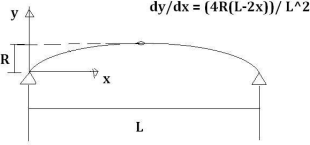Search IntMath
Close

# Length of a parabola? [Solved!]

### My question

What is the length of the parabola where dy/dx = (4Rx(L-2x)) / L^2? Here's the diagram:### Relevant page

5. Integration: Other Trigonometric Forms

### What I've done so far

I tried to use the arc length formula but there were too many letters in the problem.

X

What is the length of the parabola where dy/dx = (4Rx(L-2x)) / L^2? Here's the diagram:

<img src="/forum/uploads/parabola310.png" width="310" height"145" alt="parabola" />
Relevant page

<a href="/methods-integration/5-integration-other-trigonometric-forms.php">5. Integration: Other Trigonometric Forms</a>

What I've done so far

I tried to use the arc length formula but there were too many letters in the problem.

## Re: Length of a parabola?

Hi again

You are encouraged to use the math entry system so it's easier for you (and us) to read your math.

At least show us your first steps so we can guide you better.

Gentle warning: This has a really complicated solution. We'll get started and I'll stop you before it's too late.

X

Hi again

You are encouraged to use the math entry system so it's easier for you (and us) to read your math.

At least show us your first steps so we can guide you better.

<b>Gentle warning:</b> This has a really complicated solution. We'll get started and I'll stop you before it's too late.

## Re: Length of a parabola?

I get it a bit more now. They are already giving me the dy/dx in the question. Here's what I've got:

s=inta^b sqrt(1+(dy/dx)^2)

s=inta^b sqrt(1+((4R(L-2x))/L^2)^2)

s=inta^b sqrt(1+((16R^2(L^2-4Lx+4x^2))/L^4))

I'm stuck there

X

I get it a bit more now. They are already giving me the dy/dx in the question. Here's what I've got:

s=inta^b sqrt(1+(dy/dx)^2)

s=inta^b sqrt(1+((4R(L-2x))/L^2)^2)

s=inta^b sqrt(1+((16R^2(L^2-4Lx+4x^2))/L^4))

I'm stuck there

## Re: Length of a parabola?

Get everything under the square root, over L^4

X

Get everything under the square root, over L^4

## Re: Length of a parabola?

OK.

s=inta^b sqrt( (L^4+16R^2(L^2-4Lx+4x^2))/L^4)

=1/(L^2) inta^b sqrt( L^4+16R^2(L^2-4Lx+4x^2))

=1/(L^2) inta^b sqrt( L^4+16R^2 L^2-64R^2Lx+ 64R^2 x^2)

I'm stuck again. And what's a and b?

X

OK.

s=inta^b sqrt( (L^4+16R^2(L^2-4Lx+4x^2))/L^4)

=1/(L^2) inta^b sqrt( L^4+16R^2(L^2-4Lx+4x^2))

=1/(L^2) inta^b sqrt( L^4+16R^2 L^2-64R^2Lx+ 64R^2 x^2)

I'm stuck again. And what's a and b?

## Re: Length of a parabola?

Yes, that's good. But that's really the limit of reasonable calculation. The integral from there is really ugly.

For a and b, go back to your diagram.

Where is the origin? What are the x-coordinates of the far left and far right points of the parabola?

X

Yes, that's good. But that's really the limit of reasonable calculation. The integral from there is really ugly.

For a and b, go back to your diagram.

Where is the origin? What are the x-coordinates of the far left and far right points of the parabola?

## Re: Length of a parabola?

a=0 and b=L, correct?

So the integral will be

s=1/(L^2) int0^L sqrt( L^4+16R^2 L^2-64R^2Lx+ 64R^2 x^2)

If we have values for R and L, can it be done?

X

a=0 and b=L, correct?

So the integral will be

s=1/(L^2) int0^L sqrt( L^4+16R^2 L^2-64R^2Lx+ 64R^2 x^2)

If we have values for R and L, can it be done?

## Re: Length of a parabola?

I put it into Wolfram|Alpha with values L=10 and R=3. The answer was 12.04.

You can see the numeric solution here:

Numeric solution with L=10 and R=3

and the (horrible) algebraic answer here:

integral 1/L^2 (sqrt( L^4+16*R^2*L^2-64*R^2*L*x+ 64*R^2*x^2))dx - Wolfram|Alpha

Definitely not something we should be doing on paper!

X

I put it into Wolfram|Alpha with values L=10 and R=3. The answer was 12.04.

You can see the numeric solution here:

<a href="http://www.wolframalpha.com/input/?i=integral+from+0+to+10+0.01*%28sqrt%28+L^4%2B16*R^2*L^2-64*R^2*L*x%2B+64*R^2*x^2%29%29dx+where+L+%3D+10+and+R+%3D+3">Numeric solution with L=10 and R=3</a>

and the (horrible) algebraic answer here:

<a href="http://www.wolframalpha.com/input/?i=integral+1%2FL^2+%28sqrt%28+L^4%2B16*R^2*L^2-64*R^2*L*x%2B+64*R^2*x^2%29%29dx"> integral 1&#x2f;L&#x5e;2 &#x28;sqrt&#x28; L&#x5e;4&#x2b;16&#x2a;R&#x5e;2&#x2a;L&#x5e;2-64&#x2a;R&#x5e;2&#x2a;L&#x2a;x&#x2b; 64&#x2a;R&#x5e;2&#x2a;x&#x5e;2&#x29;&#x29;dx - Wolfram|Alpha</a>

Definitely not something we should be doing on paper!

## Re: Length of a parabola?

I agree!

Thanks a lot.

X

I agree!

Thanks a lot.

You need to be logged in to reply.

## Related Applications of Integration questions

• Re: Catenary Equation [Pending...]
Nevermind! I ended up being able to solve it
• Shell Method [Solved!]
URL:Shell Method | Brilliant Math & Science Wiki Under the section, "When to use the shell...
• Applications of Integrations #11 [Solved!]
I haven't had calculus in many years but I like to work problems every now...
• Finding volume using shells [Solved!]
Exercise 2: The area between the curve y = 1/x, the y-axis and the lines y...
• How to transform the differential equation? [Solved!]
Dear friends, I met a problem. I don't know how to transform the equation (13) into...